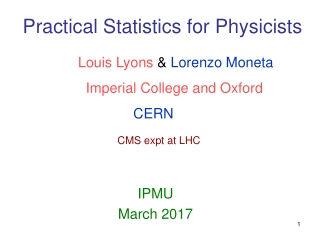DownloadDownload PresentationPractical Statistics for Physicists

# Practical Statistics for Physicists

Télécharger la présentation## Practical Statistics for Physicists

- - - - - - - - - - - - - - - - - - - - - - - - - - - E N D - - - - - - - - - - - - - - - - - - - - - - - - - - -
##### Presentation Transcript

1. Louis Lyons & Lorenzo Moneta Imperial College and Oxford CERN CMS expt at LHC Practical Statistics for Physicists IPMU March 2017

2. Learning to love the Covariance Matrix • Introduction via 2-D Gaussian • Understanding covariance • Using the covariance matrix Combining correlated measurements • Estimating the covariance matrix

3. y = 1 exp{-(x-µ)2/(22)} 2  Reminder of 1-D Gaussian or Normal

4. Correlations Basic issue: For 1 parameter, quote value and correlation For 2 (or more) parameters, (e.g. gradient and intercept of straight line fit) quote values + uncertainties + correlations Just as the concept of variance for single variable is more general than Gaussian distribution, so correlation in more variables does not require multi-dim Gaussian But more simple to introduce concept this way

5. Element Eij - <(xi – xi) (xj – xj)> Diagonal Eij = variances Off-diagonal Eij = covariances

6. Towards the Covariance Matrix

7. Inverse Covariance Matrix Covariance Matrix

8. Inverse Covariance Covariance Matrix Matrix = σx2 = Eigenvalue of covariance matrix

9. Covariance matrix

10. USING THE COVARIANCE MATRIX (i) Function of variables y = y(xa, xb) Given xa, xb covariance matrix, what is σy? Differentiate, square and average:- Covariance Matrix

11. ~ New Covariance T Old Covariance Transform matrix matrix matrix T ~ Ex = TEpT BEWARE !

12. Example from Particle Physics Track’s covariance matrix (centre of track)

13. Examples of correlated variables

14. Using the Covariance Matrix: Combining results Inverse covariance matrix

15. Small combined uncertainty Example: Chi-sq Lecture xbest outside x1 x2 ybest outside y1  y2

16. b y a x

17. ESTIMATING THE COVARIANCE MATRIX • Estimate Uncertainties • Estimate Correlations • (Usually easier if ρ =0 or 1} • 2) For independent sources of uncertainties, • add covariance matrices • e.g. MW from WW  4 Jets • and WW  JJ+lepton + ν • E= (MW)1, (MW)2 Covariance Matrix

18. Conclusion Covariance matrix formalism makes life easy when correlations are relevant